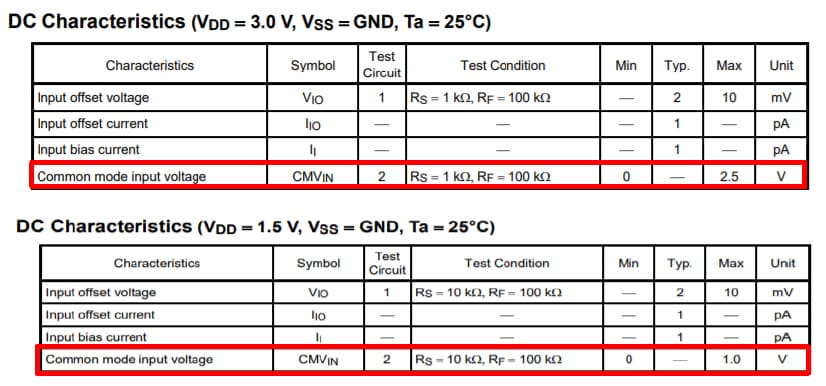# Are there any considerations for using an op-amp at low voltage?Excerpt from the DC Electrical Characteristics table shown in the datasheet for the TC75S51

When operating an op-amp at low voltage, you should take common-mode input voltage, a reduction in the signal-to-noise (S/N) ratio, and a slew rate into consideration. In particular, common-mode input voltage requires careful consideration.

1.Common-mode input voltage (CMVIN)
Check the datasheet for the common-mode input voltage range (i.e., the input voltage range in which an op-amp produces a normal output).
A non-rail-to-rail op-amp does not function properly when an input voltage is in the range between the maximum common-mode input voltage and VDD. This range is almost constant regardless of the supply voltage.
As an example, the following shows part of the DC Electrical Characteristics table given in the datasheet for the TC75S51 op-amp.
The input voltage range in which this op-amp cannot be used is fixed at 0.5 V whether VDD is 3 V or 5 V. When VDD = 1.5 V, it can be used with an input voltage in the range from GND to 1 V. As demonstrated by this example, the ratio of the valid input voltage range to VDD decreases as VDD decreases.
If the common-mode input voltage of a typical op-amp does not meet your requirement, use a rail-to-rail op-amp.

2.Reduction in the S/N ratio
This issue is related to the common-mode input voltage. The maximum input voltage that can be applied to an op-amp decreases as its supply voltage decreases. Therefore, assuming that the amount of noise applied to an op-amp remains constant, the S/N ratio decreases with the supply voltage. However, you do not need to be concerned about the deterioration of the S/N ratio if the input signal level is low and there is no need to reduce the signal amplitude.
If the S/N ratio is unsatisfactory, consider using a low-noise op-amp.

3.Reduction in the slew rate
The predominant factors that determine the slew rate of an op-amp are the parasitic capacitance of its internal signal lines and the amount of current that flows to charge and discharge this parasitic capacitance. As the supply voltage decreases, the internal current tends to decrease. Although op-amps are designed in such a manner that their internal current is not affected by the supply voltage, it still varies slightly with the supply voltage. Therefore, the slew rate tends to decrease with the supply voltage.
If the slew rate is unsatisfactory, consider using an op-amp with a high slew rate.